Electron. J. Diff. Eqns., Vol. 2005(2005), No. 79, pp. 1-25.

### Solutions approaching polynomials at infinity to nonlinear ordinary differential equations Christos G. Philos, Panagiotis Ch. Tsamatos

Abstract:
This paper concerns the solutions approaching polynomials atto-th order (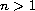) nonlinear ordinary differential equations, in which the nonlinear term depends on timeand on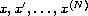, whereis the unknown function and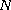is an integer with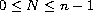. For each given integerwith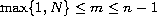, conditions are given which guarantee that, for any real polynomial of degree at most, there exists a solution that is asymptotic atto this polynomial. Sufficient conditions are also presented for every solution to be asymptotic atto a real polynomial of degree at most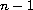. The results obtained extend those by the authors and by Purnaras  concerning the particular case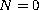.

Submitted February 13, 2005. Published July 11, 2005.
Math Subject Classifications: 34E05, 34E10, 34D05
Key Words: Nonlinear differential equation; asymptotic properties; asymptotic expansions; asymptotic to polynomials solutions.

Show me the PDF file (337K), TEX file, and other files for this article.Christos G. Philos Department of Mathematics University of Ioannina P. O. Box 1186, 451 10 Ioannina, Greece email: cphilos@cc.uoi.gr P. Ch. Tsamatos Department of Mathematics University of Ioannina 451 10 Ioannina, Greece email: ptsamato@cc.uoi.gr